Anzeige

# JAVA - Please complete the below method Ascii2Integer() USING RECURSIO.docx

20. Feb 2023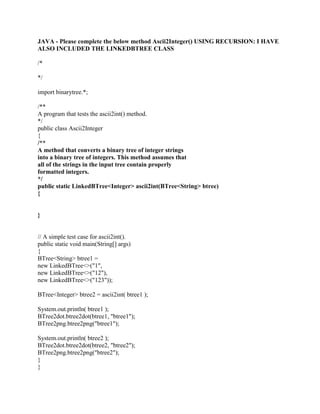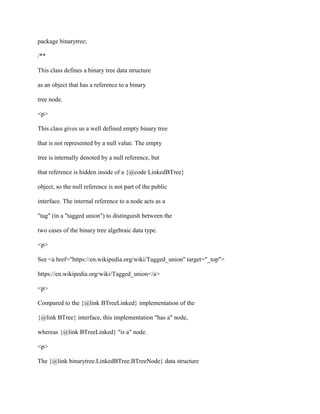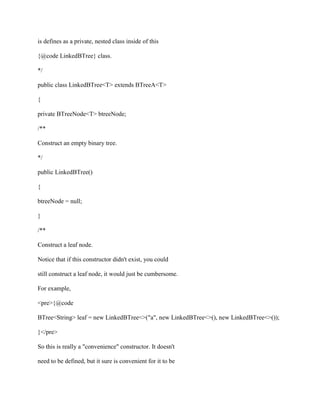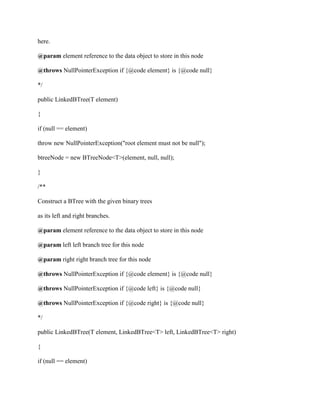Anzeige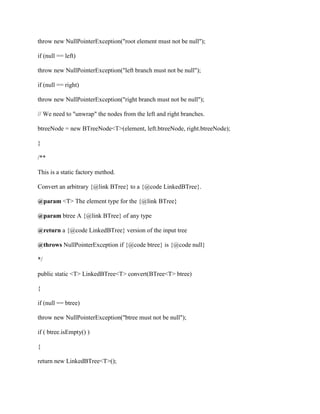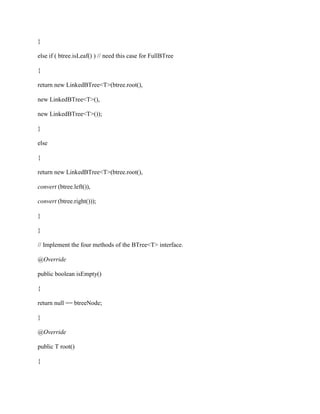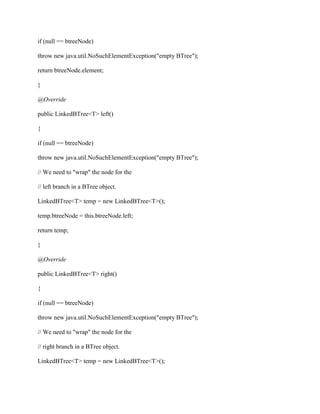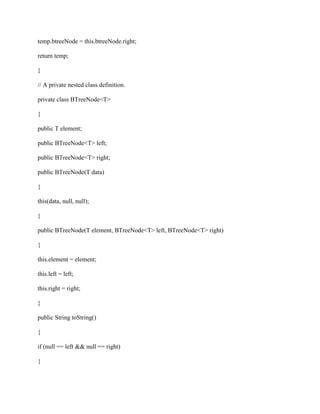Nächste SlideShareFor this project, write a program that stores integers in a binary.docx
1 von 9
Anzeige

### JAVA - Please complete the below method Ascii2Integer() USING RECURSIO.docx

1. JAVA - Please complete the below method Ascii2Integer() USING RECURSION: I HAVE ALSO INCLUDED THE LINKEDBTREE CLASS /* */ import binarytree.*; /** A program that tests the ascii2int() method. */ public class Ascii2Integer { /** A method that converts a binary tree of integer strings into a binary tree of integers. This method assumes that all of the strings in the input tree contain properly formatted integers. */ public static LinkedBTree<Integer> ascii2int(BTree<String> btree) { } // A simple test case for ascii2int(). public static void main(String[] args) { BTree<String> btree1 = new LinkedBTree<>("1", new LinkedBTree<>("12"), new LinkedBTree<>("123")); BTree<Integer> btree2 = ascii2int( btree1 ); System.out.println( btree1 ); BTree2dot.btree2dot(btree1, "btree1"); BTree2png.btree2png("btree1"); System.out.println( btree2 ); BTree2dot.btree2dot(btree2, "btree2"); BTree2png.btree2png("btree2"); } }
2. package binarytree; /** This class defines a binary tree data structure as an object that has a reference to a binary tree node. <p> This class gives us a well defined empty binary tree that is not represented by a null value. The empty tree is internally denoted by a null reference, but that reference is hidden inside of a {@code LinkedBTree} object, so the null reference is not part of the public interface. The internal reference to a node acts as a "tag" (in a "tagged union") to distinguish between the two cases of the binary tree algebraic data type. <p> See <a href="https://en.wikipedia.org/wiki/Tagged_union" target="_top"> https://en.wikipedia.org/wiki/Tagged_union</a> <p> Compared to the {@link BTreeLinked} implementation of the {@link BTree} interface, this implementation "has a" node, whereas {@link BTreeLinked} "is a" node. <p> The {@link binarytree.LinkedBTree.BTreeNode} data structure
3. is defines as a private, nested class inside of this {@code LinkedBTree} class. */ public class LinkedBTree<T> extends BTreeA<T> { private BTreeNode<T> btreeNode; /** Construct an empty binary tree. */ public LinkedBTree() { btreeNode = null; } /** Construct a leaf node. Notice that if this constructor didn't exist, you could still construct a leaf node, it would just be cumbersome. For example, <pre>{@code BTree<String> leaf = new LinkedBTree<>("a", new LinkedBTree<>(), new LinkedBTree<>()); }</pre> So this is really a "convenience" constructor. It doesn't need to be defined, but it sure is convenient for it to be
4. here. @param element reference to the data object to store in this node @throws NullPointerException if {@code element} is {@code null} */ public LinkedBTree(T element) { if (null == element) throw new NullPointerException("root element must not be null"); btreeNode = new BTreeNode<T>(element, null, null); } /** Construct a BTree with the given binary trees as its left and right branches. @param element reference to the data object to store in this node @param left left branch tree for this node @param right right branch tree for this node @throws NullPointerException if {@code element} is {@code null} @throws NullPointerException if {@code left} is {@code null} @throws NullPointerException if {@code right} is {@code null} */ public LinkedBTree(T element, LinkedBTree<T> left, LinkedBTree<T> right) { if (null == element)
5. throw new NullPointerException("root element must not be null"); if (null == left) throw new NullPointerException("left branch must not be null"); if (null == right) throw new NullPointerException("right branch must not be null"); // We need to "unwrap" the nodes from the left and right branches. btreeNode = new BTreeNode<T>(element, left.btreeNode, right.btreeNode); } /** This is a static factory method. Convert an arbitrary {@link BTree} to a {@code LinkedBTree}. @param <T> The element type for the {@link BTree} @param btree A {@link BTree} of any type @return a {@code LinkedBTree} version of the input tree @throws NullPointerException if {@code btree} is {@code null} */ public static <T> LinkedBTree<T> convert(BTree<T> btree) { if (null == btree) throw new NullPointerException("btree must not be null"); if ( btree.isEmpty() ) { return new LinkedBTree<T>();
6. } else if ( btree.isLeaf() ) // need this case for FullBTree { return new LinkedBTree<T>(btree.root(), new LinkedBTree<T>(), new LinkedBTree<T>()); } else { return new LinkedBTree<T>(btree.root(), convert (btree.left()), convert (btree.right())); } } // Implement the four methods of the BTree<T> interface. @Override public boolean isEmpty() { return null == btreeNode; } @Override public T root() {
7. if (null == btreeNode) throw new java.util.NoSuchElementException("empty BTree"); return btreeNode.element; } @Override public LinkedBTree<T> left() { if (null == btreeNode) throw new java.util.NoSuchElementException("empty BTree"); // We need to "wrap" the node for the // left branch in a BTree object. LinkedBTree<T> temp = new LinkedBTree<T>(); temp.btreeNode = this.btreeNode.left; return temp; } @Override public LinkedBTree<T> right() { if (null == btreeNode) throw new java.util.NoSuchElementException("empty BTree"); // We need to "wrap" the node for the // right branch in a BTree object. LinkedBTree<T> temp = new LinkedBTree<T>();
8. temp.btreeNode = this.btreeNode.right; return temp; } // A private nested class definition. private class BTreeNode<T> { public T element; public BTreeNode<T> left; public BTreeNode<T> right; public BTreeNode(T data) { this(data, null, null); } public BTreeNode(T element, BTreeNode<T> left, BTreeNode<T> right) { this.element = element; this.left = left; this.right = right; } public String toString() { if (null == left && null == right) {
9. return element.toString(); } else { String result = "(" + element; result += " "; result += (null == left) ? "()" : left; // recursion result += " "; result += (null == right) ? "()" : right; // recursion result += ")"; return result; } } }//BTreeNode }
Anzeige